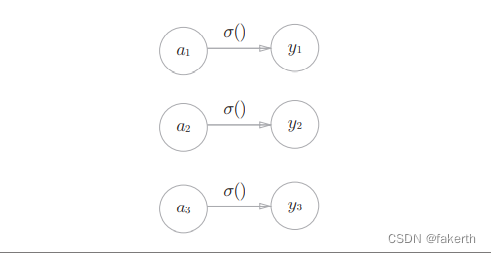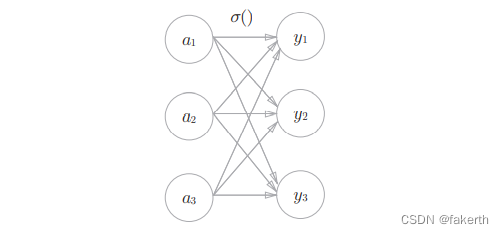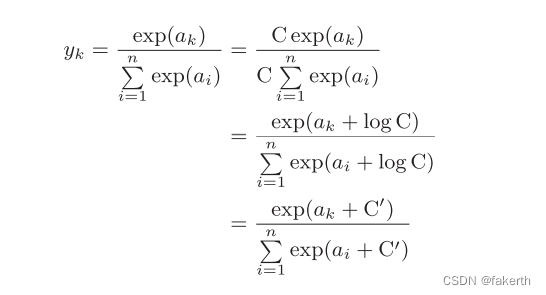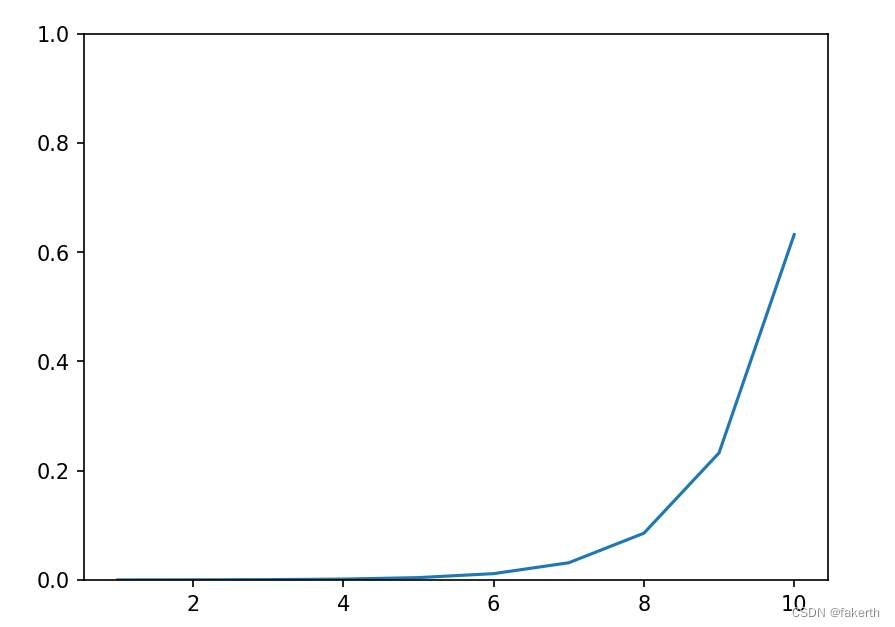#### 恒等函数#### softmax函数

softmax函数可以用下面的表达式表示：exp(x)是表示ex的指数函数（e是纳皮尔常数2.7182 …）。上式表示假设输出层共有n个神经元，计算第k个神经元的输出yk。softmax函数的分子是输入信号ak的指数函数，分母是所有输入信号的指数函数的和。``````def softmax(a):
exp_a = np.exp(a)
sum_exp_a = np.sum(exp_a)
y = exp_a / sum_exp_a
return y
``````

softmax函数的实现中要进行指数函数的运算，但是此时指数函数的值很容易变得非常大，会出现溢出问题。e100会变成一个后面有40多个0的超大值，e1000的结果会返回一个表示无穷大的inf。如果在这些超大值之间进行除法运算，结果会出现“不确定”的情况。所以我们需要对其进行改进：``````import numpy as np
import matplotlib.pylab as plt

def softmax(a):
c = np.max(a)
exp_a = np.exp(a - c)  # 溢出对策
sum_exp_a = np.sum(exp_a)
y = exp_a / sum_exp_a
return y

x = np.arange(1, 11, 1)
y = softmax(x)
plt.ylim(0, 1)  # 指定y轴的范围
plt.plot(x, y)
plt.show()
``````